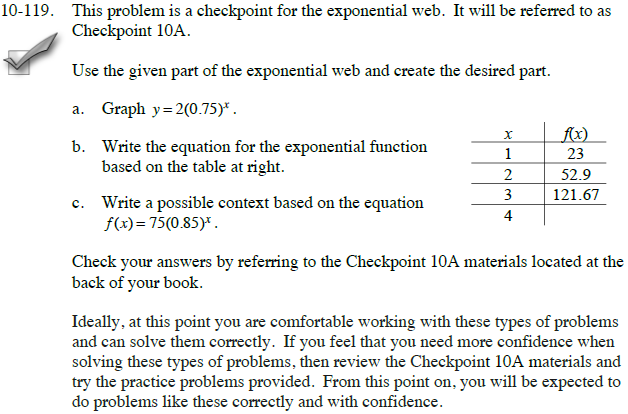### Home > CCA > Chapter 10 > Lesson 10.3.1 > Problem10-119

10-119.
1.This problem is a checkpoint for the exponential web. It will be referred to as Checkpoint 10A.

2. Use the given part of the exponential web and create the desired part. Homework Help ✎

1.  x f(x) 1 23 2 52.9 3 121.67 4

Graph y = 2(0.75)x.

2. Write the equation for the exponential function based on the table at right

3. Write a possible context based on the equation f(x) = 75(0.85)x.

Check your answers by referring to the Checkpoint 10A materials.

3. Ideally, at this point you are comfortable working with these types of problems and can solve them correctly. If you feel that you need more confidence when solving these types of problems, then review the Checkpoint 10A materials and try the practice problems provided. From this point on, you will be expected to do problems like these correctly and with confidence.Answers and extra practice are located in the back of your printed textbook or in the Reference Tab of your eBook.
If you have an eBook for CCA, login and then click the following link: Checkpoint 10A: The Exponential Web